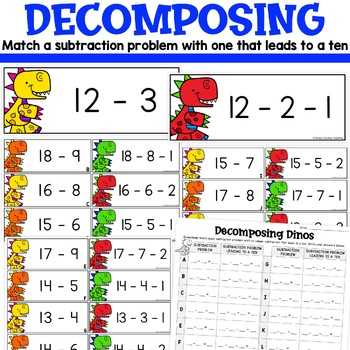Rated 4.84 out of 5, based on 19 reviews
19 Ratings;
1st
Subjects
Resource Type
Standards
Formats Included
• PDF
Pages
26 pages
Report this resource to TPT

#### What educators are saying

Loved that these centers are aligned by standards. They were easy to print laminate and go! The students enjoyed them as well.
##### Also included in
1. These are 1st grade math centers and math games for every 1st grade skill and standard. That's right! For each skill, you will access 3-5 math centers or math games that your students will love!2nd Grade Math Centers: Find them for every standard here!These 1st grade math centers include board games
Price \$49.00Original Price \$84.00Save \$35.00

### Description

Practice first grade addition and subtraction facts with these math centers! They align with 1st grade Common Core standard 1.OA.6 for students to work on subtraction with decomposing a number leading to a 10, counting on, and making ten. They consist of card games, clip cards, matching activities, and more! All facts are within 20. They are designed for you to use in your math centers and rotations.

Included are 4 different math stations for 1st grade addition & subtraction facts:

1. Decomposing Dinos: Students will match the subtraction fact with the subtraction problem that leads to a ten.
2. Feed the Monster: Students will play a fun, monster-themed card game where they will practice their subtraction facts.
3. Coffee Shop Matching: Students will match two doubles plus one facts and their sums.
4. Clip Cards: Students practice addition and subtraction facts by finding the correct match for each card.

Total Pages
26 pages
N/A
Teaching Duration
N/A
Report this resource to TPT
Reported resources will be reviewed by our team. Report this resource to let us know if this resource violates TPT’s content guidelines.

### Standards

to see state-specific standards (only available in the US).
Add and subtract within 20, demonstrating fluency for addition and subtraction within 10. Use strategies such as counting on; making ten (e.g., 8 + 6 = 8 + 2 + 4 = 10 + 4 = 14); decomposing a number leading to a ten (e.g., 13 - 4 = 13 - 3 - 1 = 10 - 1 = 9); using the relationship between addition and subtraction (e.g., knowing that 8 + 4 = 12, one knows 12 - 8 = 4); and creating equivalent but easier or known sums (e.g., adding 6 + 7 by creating the known equivalent 6 + 6 + 1 = 12 + 1 = 13).